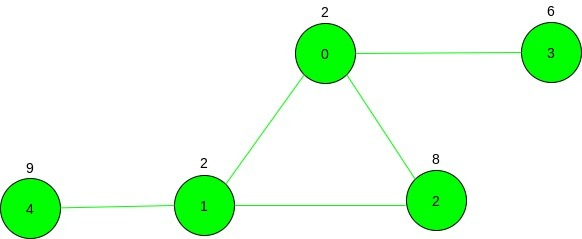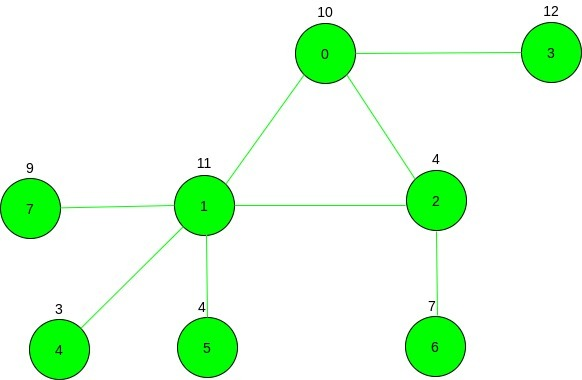# Maximum cost path in an Undirected Graph such that no edge is visited twice in a row

Given an undirected graph having N vertices and M edges and each vertex is associated with a cost and a source vertex S is given. The task is to find the maximum cost path from source vertex S such that no edge is visited consecutively 2 or more times.

Examples:

Input: N = 5, M = 5, source = 1, cost[] = {2, 2, 8, 6, 9}, Below is the given graph:Output: 21
Explanation:
The maximum cost path matrix is given as:
1 -> 2 -> 0 -> 1 -> 4
Cost = 2 + 8 + 2 + 2 + 9 = 21

Input: N = 8, M = 8, source = 3, cost[] = {10, 11, 4, 12, 3, 4, 7, 9}Output: 46
Explanation:
The maximum cost path matrix is given as:
3 -> 0 -> 2 -> 1 -> 7

## Recommended: Please try your approach on {IDE} first, before moving on to the solution.

Approach: The idea is to check if there exists a loop exists in the graph, then all vertices of the loop needs to be traversed and then traverse graph towards the leaf nodes with maximum cost. And if loop does not exists then then the problem statement converts to find maximum cost path in any directed graph.
Below are the declaration used in the program:

• dp[i]: stores the total cost to traverse the node ‘i’ and all it’s children node.
• vis[i]: marks the nodes which have been visited.
• canTake: stores the resultant sum of all node of maximum cost path excluding the leaf vertex and its children node, if it exists.
• best: stores the cost of maximum cost leaf node and its children node(if it exists).
• check: boolean variable used as a flag to find loop in the graph, its value changes to 0 when loop is found.

Below are the steps:

1. Perform DFS traversal with flag variable check set to ‘1’ initially denoting no loop found.
2. Simultaneously build the dp[] for each node with the maximum cost updated till that traversed node.
3. If adjacent node is found to be already visited and it is not parent node then loop is found and set value of check to 0.
4. Add cost of all nodes of the loop to canTake.
5. After traversing adjacent nodes of the traversing node, no loop is found, then it represent cost of path leading from loop to leaf vertex and update best to dp[i] if dp[i] is greater than best.
6. After traversal of the graph, print the sum of canTake and best.

Below is the implementation of the above approach:

## C++

 `// C++ program for the above approach ` `#include ` `using` `namespace` `std; ` `const` `int` `N = 100000; ` ` `  `// To store the resulting ` `// sum of the cost ` `int` `canTake; ` ` `  `// To store largest ` `// cost leaf vertex ` `int` `best; ` ` `  `int` `dp[N]; ` `bool` `vis[N]; ` ` `  `// DFS Traversal to find the update ` `// the maximum cost of from any ` `// node to leaf ` `int` `dfs(vector >& g, ` `        ``int``* cost, ``int` `u, ``int` `pre) ` `{ ` ` `  `    ``// Mark vertex as visited ` `    ``vis[u] = ``true``; ` ` `  `    ``// Store vertex initial cost ` `    ``dp[u] = cost[u]; ` ` `  `    ``// Initially assuming edge ` `    ``// not to be traversed ` `    ``bool` `check = 1; ` ` `  `    ``int` `cur = cost[u]; ` `    ``for` `(``auto``& x : g[u]) { ` ` `  `        ``// Back edge found so, ` `        ``// edge can be part of ` `        ``// traversal ` `        ``if` `(vis[x] && x != pre) { ` `            ``check = 0; ` `        ``} ` ` `  `        ``// New vertex is found ` `        ``else` `if` `(!vis[x]) { ` ` `  `            ``// Bitwise AND the current ` `            ``// check with the returned ` `            ``// check by the previous ` `            ``// DFS Call ` `            ``check &= dfs(g, cost, x, u); ` ` `  `            ``// Adds parent and its ` `            ``// children cost ` `            ``cur = max(cur, ` `                      ``cost[u] + dp[x]); ` `        ``} ` `    ``} ` ` `  `    ``// Updates total cost of parent ` `    ``// including child nodes ` `    ``dp[u] = cur; ` ` `  `    ``// Edge is part of the cycle ` `    ``if` `(!check) { ` ` `  `        ``// Add cost of vertex ` `        ``// to the answer ` `        ``canTake += cost[u]; ` `    ``} ` `    ``else` `{ ` ` `  `        ``// Updates the largest ` `        ``// cost leaf vertex ` `        ``best = max(best, dp[u]); ` `    ``} ` ` `  `    ``return` `check; ` `} ` ` `  `// Function to find the maximum cost ` `// from source vertex such that no ` `// two edges is traversed twice ` `int` `FindMaxCost(vector >& g, ` `                ``int``* cost, ``int` `source) ` `{ ` `    ``// DFS Call ` `    ``dfs(g, cost, source, -1); ` ` `  `    ``// Print the maximum cost ` `    ``cout << canTake + best; ` `} ` ` `  `// Driver Code ` `int` `main() ` `{ ` `    ``int` `n = 5, m = 5; ` ` `  `    ``// Cost Array ` `    ``int` `cost[] = { 2, 2, 8, 6, 9 }; ` ` `  `    ``vector > g(n); ` ` `  `    ``// Given Graph ` `    ``g.push_back(1); ` `    ``g.push_back(0); ` `    ``g.push_back(2); ` `    ``g.push_back(0); ` `    ``g.push_back(3); ` `    ``g.push_back(0); ` `    ``g.push_back(2); ` `    ``g.push_back(1); ` `    ``g.push_back(4); ` `    ``g.push_back(1); ` ` `  `    ``// Given Source Node ` `    ``int` `source = 1; ` ` `  `    ``// Function Call ` `    ``FindMaxCost(g, cost, source); ` `    ``return` `0; ` `} `

Output:

```21
```

Time Complexity: O(N + M) where N is number of vertices and M is number of edges
Auxillary Space: O(N + M) where N is number of vertices and M is number of edges

Attention reader! Don’t stop learning now. Get hold of all the important DSA concepts with the DSA Self Paced Course at a student-friendly price and become industry ready.

My Personal Notes arrow_drop_upCheck out this Author's contributed articles.

If you like GeeksforGeeks and would like to contribute, you can also write an article using contribute.geeksforgeeks.org or mail your article to contribute@geeksforgeeks.org. See your article appearing on the GeeksforGeeks main page and help other Geeks.

Please Improve this article if you find anything incorrect by clicking on the "Improve Article" button below.